# NCERT Solution, Tenths And Hundredths, class 5, Mathematics Notes | Study Mathematics for Class 5: NCERT - Class 5

## Class 5: NCERT Solution, Tenths And Hundredths, class 5, Mathematics Notes | Study Mathematics for Class 5: NCERT - Class 5

The document NCERT Solution, Tenths And Hundredths, class 5, Mathematics Notes | Study Mathematics for Class 5: NCERT - Class 5 is a part of the Class 5 Course Mathematics for Class 5: NCERT.
All you need of Class 5 at this link: Class 5

NCERT Solution, Tenths And Hundredths, class 5, Mathematics

1. Length of the nail …. Cm and …. Mm or …. Cm.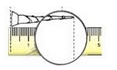Ans.

Length of the nail 2 cm and 9 mm or 2.9 cm.

2. The length of this lady’s finger (bhindi) is …. cm and …. Mm. We can also write it as .. mm.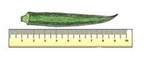Ans.

The length of this lady’s finger is 8 cm and 3 mm. We can also write it as 8.3 cm.

3. Using the scale on this page find the difference in length between candle 1 and candle 3?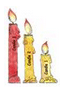Ans.

Length of Length in cm and mmLength in cm.Candle 12 cm and 9 mm2.9 cmFlame 11 cm and 3 mm1.3 cmCandle 24 cm and 9 mm4.9 cmFlame 21 cm and 6 mm1.6 cmCandle 36 cm and 0 mm6 cmFlame 31 cm and 5 mm1.5 cm

4. Our Eyes Get Confused?

Which line is longer? A or B? Measure each line and writes how long it is in centimetres. How good is your guess?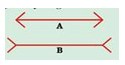Ans.

I think line B is longer than A. On Measuring: line (A) = 4.6 cm and Line (B) = 4.6 cm.

My guess is incorrect.

5. Which line is longer? C or D? Measure each line. How good is your guess?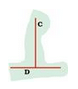Ans.

Line (C) appears to be longer than the line (D). On measuring: Line (C) = 3.2 cm and Line (D) = 3.2 cm. My guess in incorrect.

6. At the market

(a) How many paise does a matchbox cost?

Ans.

A matchbox costs 50 paise.

(b) How many match-box can be got for Rs. 2.50?

Ans.

Five matchboxes can be bought for Rs. 2.50.

(c) How many rupees does the soap cost?

Ans.

A soap costs Rs. 8.75.

(d) Arun wanted to buy a soap. He has a five-rupees coin. 2 one-rupee coins and 4 half-rupee coin. Write in rupees what money he will get back?

Ans.

Total money with Arun = 1 x Rs. 5 + 2 x Re 1 + 4 x Re. 0.5

= Rs. 5 + Rs. 2 + Rs. 2 = Rs. 9

Cost of a soap = Rs. 8.75

Arun will get back = Rs. 9 – Rs. 8.75

= 900 paise – 875 paise

= 25 paise = Re 0.25

(e)(1) An egg costs two and a half rupees. How much will one and a half dozen cost?

Ans.

(1) One dozen =12 pieces

One and a half dozen = (12+6) = 18 pieces

Cost of one egg = Rs. 2.50

Cost of 18 eggs = Rs. (18 x 2.50)

= Rs. 18 x 2 + Rs. 18 x 0.50

= Rs. 36 + Rs. 9 = Rs. 45

(2) How many pens can Kannan buy? How much money is left?

Ans. (2) Money left after purchase of one and a half dozen eggs

= Total money – Money spent

= Rs. 60 – Rs. 45

= Rs. 15

Cost of a pen = Rs. 6.50

Since 1 x Rs. 6.50 = Rs. 6.50, 2 x Rs. 6.50 = Rs. 13

3 x Rs. 6.50 = Rs. 19.50

Money left with Kanna = Rs. 15- Rs. 13 = Rs. 2

(f) The price of two pens is Rs …… Can she buy two pens?

Ans. Price of 1 pen = Rs. 6.50

Price of 2 pens = Rs. (2 x6.50) = Rs. 13

So, she can buy 2 pens with rupees 13.

7. Colourful Design: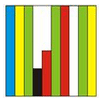(a) What part of this sheet is coloured blue?

Ans. (a)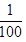part of the given sheet is coloured blue.

(b) Which part of the sheet is green?

Ans.(b) 3/10 part of the given sheet is green.

(c) Which colour covers 0.2 of the sheet?

Ans. (c) Black colour covers 0.2 of the given sheet.

8.  Now look at the second sheet. Each strip is divided into 10 equal boxes. How many boxes are there in all?

(a) Is each boxpart of the sheet?

Ans. (a) Yes, each box ispart of the sheet.

(b) How many blue boxes are there?

Ans. (b) There are 10 blue boxes.

(c) Is blue equal to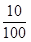of the sheet? We saw that blue is also equal toof the sheet. We wrote it as 0.1 of the sheet.

Ans. (c) Yes, blue boxes are equal toof the sheet.

(d) Can we say== 0.10 = 0.1 ?

Think: Can we write ten paise is 0.1 of a rupee?

Ans. (d) Yes, we can say== 0.10 = 0.1.

(e) How many boxes are red? What part of the sheet is this?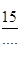Can we also write it as 0.15 of the sheet?

Ans. There are 15 red boxes. They are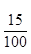part of the sheet.

It can also be written as 0.15 of the sheet.

(f) Now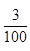of the sheet is black. We can say 0. ……. Is black.

Ans. Nowof the sheet is black. We can say 0.03 sheet is black.

(g) How many white boxes are there in the sheet?

Ans. There are 22 white boxes in the sheet.

(h) What part of the second sheet is white?

Ans. 22/100  part of the second sheet is white.

9. Sports Day The school at Malappuram has its sports day. The first five children in the Long Jump are: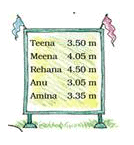(a) Who is the winner in the long jump?

Ans. (a) Rehana was the winner.

(b) Write the names of the I , II and III winners on this stand.

Ans.  I is Rehana, II is Meena and III is Teena.

(c) Do you remember that 1 metre = 100 centimetere? So one centimeter isof a metre. We also writes 1 cm as ….. m.

Ans. (c) 1 cm as 0.10 m.

10.  Money from different countries.

Have you seen any notes or coins used in any other country? Shivam Bank has a chart to show us how many Indian rupees we can get when we change the money of different countries.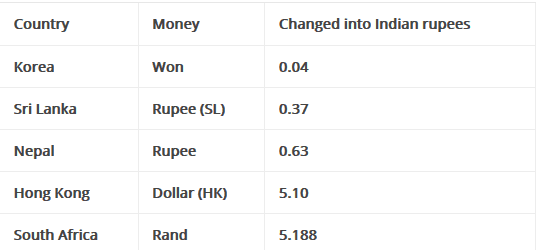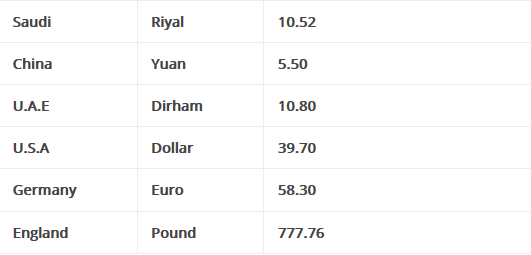(This is the rate on 15-2-2008)

(A) The money of which country will cost the most in Indian Rupees?

Ans.

England’s money will cost the most in Indian Rupees.

(B) Mithun’s uncle in America had sent hin 10 USA dollars as a gift. Mithun used 350 rupees for a school trip. How much money was left with him.

Ans.

Money received by Mithun = 10 USA Dollars

= 10 x Rs. 39.70

= 10 x Rs. 39 + 10 x 70 paise

= Rs. 390 + 700 paise

= Rs. 390 + Rs. 397

Money spend on school trip =Rs. 350

Money left with Mithun = Rs. 397- Rs. 350 = Rs. 47

(C) Majeed’s father is working in Saudi Arabia. He gets 1000, Saudi Riyal as salary. Arun’s father who is working in Sri Lanka gets 2000 Sri Lankan Rupees. Who gets more Indian Rupees.

Ans.

Majeed’s father salary = 1000 Saudi Riyal

= 1000 x Rs. 10.52

= 1000 x Rs. 10 + 1000 x 52 paise

= Rs. 10000 + 52000 paise

= Rs. 10000 + Rs. 520 = Rs. 10520

Arun’s father salary = 2000 Sri Lankan Rupees

= 2000 x Rs. 0.37

= 2000 x 37 paise

= 74000 paise = Rs. 740

(D) Leena’s aunty brought a present for her from China. It cost 30 Yuan. Find what it costs in Indian rupees.

Ans. Cost of the present = 30 Yuan

= 30 x Rs. 5.50

= 30 x Rs. 5 + 30 x 50 paise

= Rs. 150 + Rs. 15 = Rs. 165

(E) Astha wants some Hong Kong Dollars and Won.

(1) How many won can Hong Kong Dollars and Won.

Ans. Since 1 won = Re. 0.04

Multiply both sides by 100, we get

100 x 1 Won = 100 x Re. 0.04

= 100 x 4 paise

= 400 paise = Rs. 4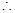For Rs.4 Astha can have 100 Won

And, for Rs.400, she can have 100x 100 = 10000 won

(2) How many Hong Kong Dollars can she changes for Rs. 508?

Ans. Since 1 Dollar (HK) = Rs. 5.10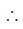For Rs. 508, Astha can have 99 Dollar and have left with Rs. 508- Rs. 504.90 i.e. Rs. 3.10 in hand.

11. Kiran went shopping with Rs. 200. Look at the bill. The shopkeeper forgot to put the point correctly in the prices. Put the point in the correct place and find out the total amount of the bill.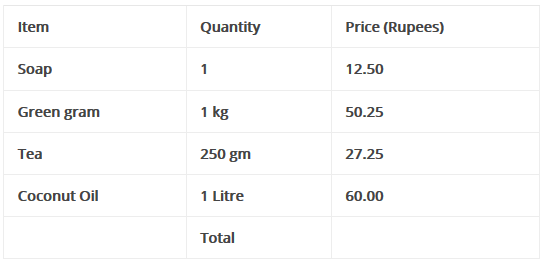Ans.

The correct bill as under: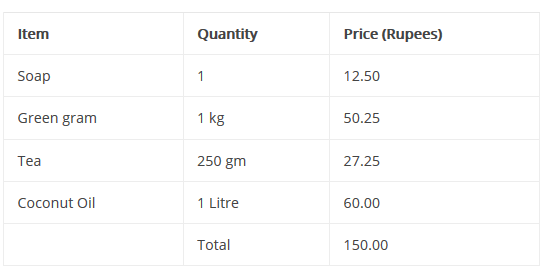12. Which city is cool?

The temperature in each city was noted at 3pm on 16 January, 2008 as tabulated below:

(a) Which place had the highest temperature at 3 pm? Which place is the coolest at that time?

Ans.

(a) Mumbai had the highest temperature at 3 pm. Srinagar had the coolest temperature at 3 pm.

(b)  How much higher is the temperature in Mumbai from that in Srinagar?

Ans.

(b) The temperature in Mumbai is higher from that in Srinagar by 35.1C. 8.1C = 27C

(c) How many degrees will the temperature need to rise for it to reach 40C in Thiruvananthapuram?

Ans. (c) There should be a rise of 40o C- 33.5C = 6.5C on temperature for it to reach 40C in Thiruvananthapuram.

(d) How much lower is the temperature of Kolkata from that in Chennai?

Ans. (d) The temperature in Kolkata is lower than in Chennai by 29.9oC – 26.6C = 3.3C.

(e) The temperature in these cities was also noted at 3 am on the same day, Look at the table and answer the questions.

Q. Which place had the lowest temperature at 3 am? Imagine yourself to be there and describe how it would feel?

Ans. Srinagar had the lowest temperature at 3 am. It was very much cold and the water n pipes froze into ice. We had worn heavy wollen clothes and had also taken the help of fire.

Q. What is the difference between the temperatures at 3 pm and 3 am in Chennai?

Ans. The difference between the temperatures at 3 pm and 3 am in Chennai is 29.9C – 21.1oC = 8.8oC.

The document NCERT Solution, Tenths And Hundredths, class 5, Mathematics Notes | Study Mathematics for Class 5: NCERT - Class 5 is a part of the Class 5 Course Mathematics for Class 5: NCERT.
All you need of Class 5 at this link: Class 5Use Code STAYHOME200 and get INR 200 additional OFF

## Mathematics for Class 5: NCERT

36 videos|44 docs|39 tests

Track your progress, build streaks, highlight & save important lessons and more!

,

,

,

,

,

,

,

,

,

,

,

,

,

,

,

,

,

,

,

,

,

,

,

,

,

,

,

,

,

,

;